# How to Find the Average in Excel?

Affiliate disclosure: In full transparency – some of the links on our website are affiliate links, if you use them to make a purchase we will earn a commission at no additional cost for you (none whatsoever!).

As soon as we hear the term “statistics”, the first thing that strikes to most of the individuals is mean, median and mode. The mean, which is often called the average, is the measure of central tendency that most people are familiar with. How to find the average is, basically the easiest out of all the measures of central tendency.

In conversational language, an average is the sum of a list of numbers divided by the number of numbers that are imbibed in that particular list. In mathematics and statistics, this would be called the arithmetic mean.

Let’s have a look at how do you find the average:

# Calculation of Mean

As discussed above, the mean is the sum of the value of each observation in a dataset divided by the number of observations. This is also known as the arithmetic average. In simple words, the sum is divided by the count. Also, learn to divide in Excel.

Example 1: Let’s have a look at the distribution:

3, 7, 5, 13, 20, 23, 39, 23, 40, 23, 14, 12, 56, 23, 29

The sum of these numbers is 330

There are fifteen numbers.

The mean is equal to 330 / 15 = 22

Thus, the mean of the above numbers is 22.

Example 2: Let’s have a look at the distribution:

9 13 9 11 9 13 11 9 10 8 11

The mean for this example is:

9+ 13+ 9+ 11+ 9+ 13+ 11+ 9+ 10+ 8+ 11= 113

There are 11 numbers.

The mean is equal to 113/11= 10.27

You can write a mean as a decimal number or round to a whole number. Thus, the mean is 11.

Example 3: Let’s have a look at how to handle negative numbers:

3, −7, 5, 15, −6

The sum of these numbers is 3 − 7 + 5 + 15 − 6 = 10

There are 5 numbers.

The mean is equal to 10 ÷ 5 = 2

Thus, the mean of the above numbers is 2.

## Options for Entering the Function

Basically, there are three options for entering the functions in Excel. These are:

1. You need to type the complete function =AVERAGE(C1:C4) into a worksheet cell.
2. You can simply, enter the function and arguments using the function’s dialog box.
3. Use Excel’s Auto Average feature in order to enter the function and arguments.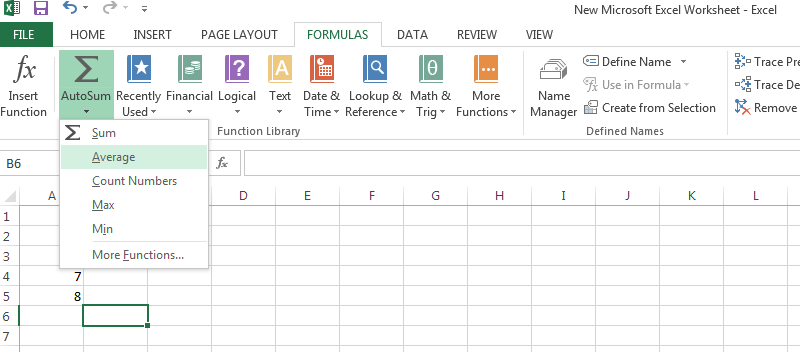Now let’s study each of them in detail-

## How to Find the Average in Excel

Microsoft Excel is an ideal program to perform any numerical analysis such as computing the mean, median, mode and range for a set of numbers. Expect for calculating range, the Excel has a built-in function of how to calculate average. Let’s have a look at the steps:

2. Now, choose the cells you want to average.
3. Make a note of their cell references, using the format A1 or a range of A1:A5. Press “Enter” to complete the formula and the mean of your numbers will appear in the cell.
4. Enter “=AVERAGE (range)” in an empty cell and replace “range” with the cells you wish to average.
5. Example- To average, the cells A1 through A5, enter “=AVERAGE(A1:A5)”6. The non- consecutive cells can be separated by including a comma. Example- “=AVERAGE(A1:A6,A9,D3)”### Function Dialog Box

Now, let’s have a look as to how the function dialog box operates, via following the enlisted steps:

1. Click on the cell, i.e. the location where the function results are displayed. Let’s say it is cell C9 in this case.
2. Click on the Formulas tab of the ribbon.
3. Choose More Functions > Statistical from the ribbon to open the function drop down list and click on AVERAGE in the list to bring up the function’s dialog box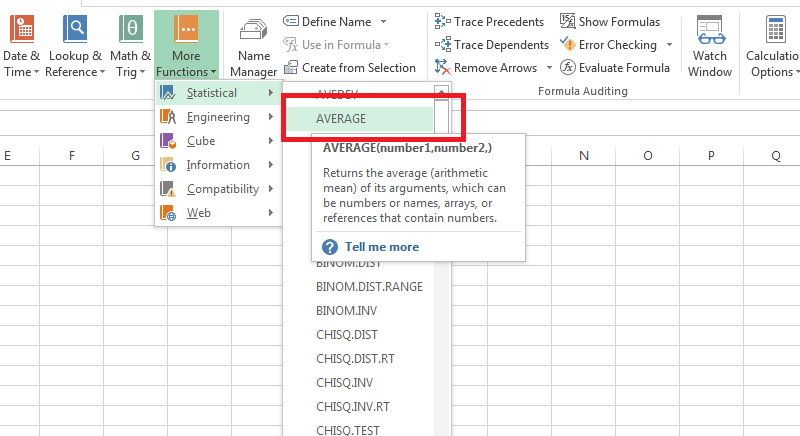4. Highlight the cells C1 to C8 to enter the range into the dialog box and click OK to close the dialog box and return to the worksheet.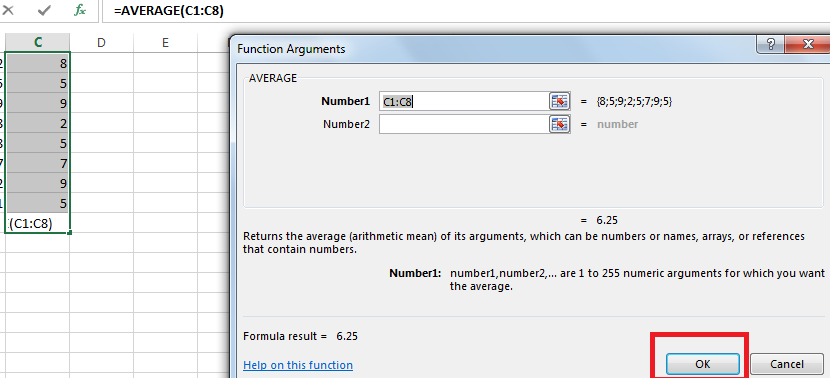5. When you click on cell C9 the complete function =AVERAGE(C1:C8) appears in the formula bar above the worksheet.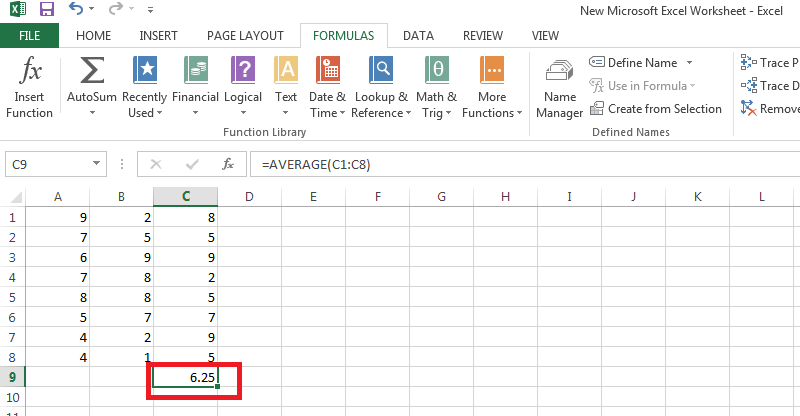### How to Calculate Weighted Average Mean

Usually, when the average is calculated, we put same weight or priority to each value, this is called un-weighted average. If you have really big data for addition, you should take a look at adding numbers in excel.

When weight is also taken into consideration while finding the average, it is known as weighted average. To find the weighted mean:

1. Multiply the numbers in your data set by the weights.

Example- Let’s say you assign equal weights to your data set of 5 numbers. Thus, the weighted mean would be:
1(*1/5) + 3(*1/5) + 5(*1/5) + 7(*1/5) + 10(*1/5) = 5.2

Note- In case, you have very high or very low values in your data set, the weighted mean may not be a good statistic to rely on.

### How to Calculate Weighted Average Mean in Excel

First of all, for how to find an average, you need to have knowledge of Excel SUMPRODUCT function. SUMPRODUCT is simply, multiplying each number with its weight and then adding all of the products together.

The formula is:

= SUMPRODUCT(<Range containing all the Elements>, <Range containing weight of all the Elements >)/ SUM(<Range containing weight of all the Elements >)

Follow the following process for the same in Excel:

1. Set up the spreadsheet and enter the formula in any empty cell.
2. Start the formula with an = sign.
3. Type SUMPRODUCT with an open parenthesis, like this =SUMPRODUCT(4. Add arguments to the function. Usually it has two arguments and separate the arguments with a comma. Let’s assume our two arguments are contained in these cells:

=SUMPRODUCT (B4:B9, C4:C9)1. Now, divide SUMPRODUCT by the SUM of the weights. Start by typing a / (forward slash) for division, and then type the SUM function:

=SUMPRODUCT(B2:B9, C2:C9)/SUM(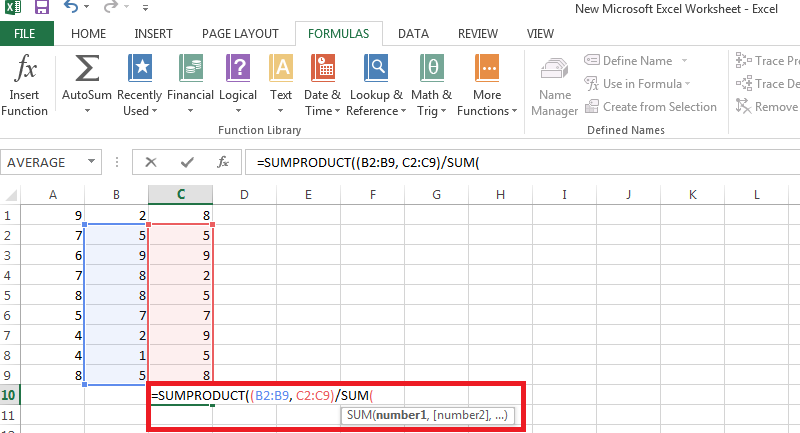1. Now, in order to close the parentheses you need one argument only. Let’s say we have C4: C9

=SUMPRODUCT(B4:B9, C4:C9)/SUM(C2:C9)1. To finish, just press Enter on your keyboard.

#### Blank Cells

Always remember that there is a difference between blank or empty cells and those containing a zero value. The blank cells are ignored by the AVERAGE function but not those containing a zero value.

Sometimes by default, Excel displays a zero in cells with a zero value. So turn off this option in order to avoid errors and inconvenience. Once this option is turned off, such cells are left blank, but are still included in average calculations.

Computing the numbers on excel is easy but to make it the numbers presentable, you may need some graphics. Here is how you can create bar graphs in excel to make the data presentable and understandable.

#### Conclusion

Working on Excel may seem tough in the beginning, but once you are eloquent about it, the work becomes comparatively simpler and easier. How to find the average is a function most widely used by professionals while making reports or during calculations.

So go ahead, and calculate the averages in a simplified way!##### arun singh

Arun Singh is a experienced server management geek and he has more than 8 years of experienced handling hosting server and he do sometimes share his knowledge in digital marketing. He currently with private company in Mumbai, India and he handles server management at BloggersIdeas.com.